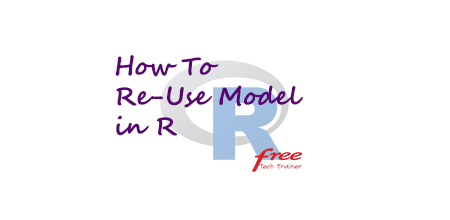# How to Reuse Model in RIn our day to day life we have to create many models or we have to work with many models. Sometimes we need to reuse those models in future. Generally if we don’t save our created objects of models we can not use those objects after closing the application. So, basic things to reuse objects in R is to save created objects before closing the application. Can we do this job in R? Yes, R has an option to do that. Today, I’ll show you how to save object of model in R in step by step with example.

Let’s create a data frame with a independent variable x of 100,000 rows applying rnorm function. Then let’s create a dependent variable y with an equation.

```#creating a data frame sal_df with independent variable x
sal_df <- data.frame(x = rnorm(100000))
sal_df <- transform(sal_df, y = 30000 + (3.6 * x) + rnorm(100000))
sal_lm <- lm(y ~ x, data = sal_df)

```

Now, it’s time to close my application. But before closing my application, let’s save the object.

```#saving the model
save(sal_lm, file = "d:/analytics_works/sal_lm.rdo")
#closing the program
q()

```

After some days, again I need to use that model to predict a newly created data frame. So, let’s load the object of previously saved object of our specific model.

```#loading previously saved model object